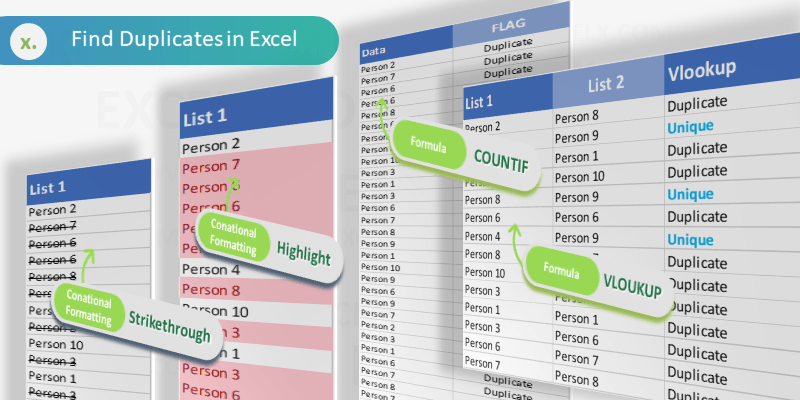It is very easy to find duplicates in Excel. We can use built in tools (Conditional Formats, Filters) or formula (COUNTIF or VLOOKUP)  to find duplicates in Excel Columns or Rows. Let us see the best and easy methods to find duplicate values in Excel.

## Find duplicates Using Conditional Formatting

We can easily identify the duplicates using Conditional Formatting Tools in Excel. We can select the range and highlight the duplicate values with color.  Following are the different methods to find duplicate entries in excel and strikethrough the duplicates using Conditional Formatting.

### Highlighting duplicate values in Excel

We can highlight the duplicate values in the Excel with specific color. We can change the fill color and font color for the duplicate values. Please follow the below steps to identify the duplicates.

• Select the required range of cell to find duplicates
• Go to ‘Home’ Tab in the Ribbon Menu
• Click on the ‘Conditional Formatting’ command
• Go to ‘Highlight Cells Rules’ and Click on ‘Duplicate Values…’
• And Choose the formatting Options from the drop down list and Click on ‘OK’

Now you can see that all the duplicate values are highlighted as shown in the screen-shot.

### Strike through duplicate values in Excel

We can strike through the duplicate values in the Excel. It is very helpful to strike out all duplicate entries in a range of cells. We can use the conditional formatting command in Excel to strike through the duplicate Cells.

• Select the required range of cell to strike through the duplicates
• Go to ‘Home’ Tab in the Ribbon
• Click on the ‘Conditional Formatting’ command
• Go to ‘Highlight Cells Rules’ and Click on ‘Duplicate Values…’
• And Choose the Custom Format from formatting Options from the drop down list
• Select the Strikethrough option from Font effects and Click on ‘OK’

Now you can see that all the duplicate values are stroked out as shown in the screen-shot.

## Formula for checking duplicates

We can create formula using COUNTIF or VLOOKUP Functions to check the duplicates in Excel. Following is the step by explanation for finding duplicate values using Excel Formula.

### Finding Duplicates using COUNTIF Formula

We can use COUNTIF Formula in Excel Cells to identify duplicates in Excel. COUNTIF formula helps to find duplicates in One Column, returns the number of occurrences of a given value. We can identify the duplicates if the Count is greater than 1. We can create new column to mark the duplicates using Excel COUNTIF Formula.

`COUNTIF(A:A,A2)`

You can see that we have enter the COUNTIF formula in Range B2 to check the total occurrences of A1 value in the entire Column A:A.This will return the frequency of each Cell value of Column A in Column B.

We can identify the duplicate Cells based on the value in the Column B. If the values is greater than 1, we confirm that the values contains duplicate entries in the specified range or column.

### Mark Duplicates and Unique Entries using Formula

We can enhance the above formula to mark all duplicates in a Column. The following formula will return ‘Duplicate’ if the cell value contains duplicate values, otherwise returns ‘Unique’.

`=IF(COUNTIF(A:A,A2)>1,"Duplicate","Unique")`

Formula will return ‘Duplicate’ in the Column B if the value repeats (count >1).

It will return ‘Unique’ in the Column B if the count is 1.

### Find duplicate values in excel using VLookup

We can use VLOOKUP formula to compare two columns (or lists) and find the duplicate values. Vlookup helps to find duplicates in Two Column and duplicate rows based on Multiple Columns. Following are steps to find duplicates using VLOOKUP function.

We have two lists in the Excel List 1 in Column A and List 2 in Column B. Let us find the duplicate values in the List 2 which are part of List 1.

`=VLOOKUP(B2,\$A\$2:\$A\$15,1,FALSE)`

VLOOKUP Formula will check for the Cell B2 value in the specified Range (A2:A5) and Returns the value if found, it will return #NA if it is not found in List 1. We can confirm if the the value is duplicated in List 1 and 2 if it returns the Value, it will be unique if it returns #NA.

#### How to find duplicate values in Excel using VlookUp?

You can use Excel formula to find the duplicate values in Excel by using vlookup formula as described below. You can use the Excel VlookUp to identify the duplicates in Excel.

`=IF(ISNA(VLOOKUP(B2,\$A\$2:\$A\$15,1,FALSE)),"Unique","Duplicate")`

We can enhance this formula to identify the duplicates and unique values in the Column 2. We can use the Excel IF and ISNA Formulas along with VLOOKUP to return the required Labels.

You can use this method to compare with other worksheets and find duplicate values in Different Excel Sheets.

#### How to find duplicate values in two columns in excel using VlookUp?

Follow the below steps to find the duplicate values in two columns in excel using VlookUp. We generally need this to compare two columns and check if a value is existing in two columns.

```=IF(IF(ISNA(VLOOKUP(C2,\$A\$2:\$A\$15,1,FALSE)),0,1)
+IF(ISNA(VLOOKUP(C2,\$B\$2:\$B\$15,1,FALSE)),0,1)=2,"Duplicated","Unique")
```

Here, we have Two Target Columns A and B , we check if the items in Column C are duplicated or not.

If you wants to compare two columns and check for values are repeated in both the columns or not, you can use this formula.